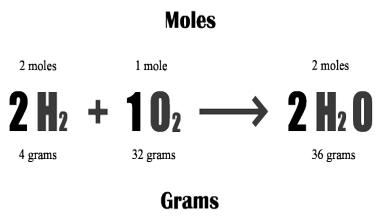Chemistry

# Introduce Mole

For practical purposes, a microscopic (i.e. how many atoms) view of substances is not useful. We need to deal in macroscopic (e.g. how many grams) quantities of elements and compounds. To do this, we introduce the mole.

One mole of a substance is the amount of substance which contains a standard number of particles (atoms, ions or molecules). This standard number is defined as the same number of particles as there are Carbon atoms in 12g of the isotope Carbon-12. This is the Avogadro number 6.022 x 1023 particles.

So 12g of Carbon-12 represents one mole (written 1 mol) of Carbon-12. It contains 6.022 x 1023 Carbon atoms. Similarly one mole of sodium atoms contains 6.022 x 1023 sodium atoms. And half a mol of Neon atoms contains 3.011 x 1023 Neon atoms.Because atoms of different elements do not have the same mass, one mole of sodium will not be as heavy as one mole of calcium. However, the mass of any substance can easily be related to the number of particles in it, as follows:

• A.M. of C = 12 and R.A.M. of He = 4
• 1 C atom is 3 times as heavy as 1 He atom
• 10 C atoms are 3 times as heavy as 10 He atoms
• 1000 C atoms are 3 times as heavy as 1000 He atoms
• 106 C atoms are 3 times as heavy as 106 He atoms